Next: Worked example 1.1: Conversion Up: Introduction Previous: Precision and significant figures

## Dimensional analysis

As we have already mentioned, length, mass, and time are three fundamentally different quantities which are measured in three completely independent units. It, therefore, makes no sense for a prospective law of physics to express an equality between (say) a length and a mass. In other words, the example law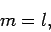(3)

whereis a mass and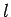is a length, cannot possibly be correct. One easy way of seeing that Eq. (3) is invalid (as a law of physics), is to note that this equation is dependent on the adopted system of units: i.e., if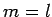in mks units, thenin fps units, because the conversion factors which must be applied to the left- and right-hand sides differ. Physicists hold very strongly to the assumption that the laws of physics possess objective reality: in other words, the laws of physics are the same for all observers. One immediate consequence of this assumption is that a law of physics must take the same form in all possible systems of units that a prospective observer might choose to employ. The only way in which this can be the case is if all laws of physics are dimensionally consistent: i.e., the quantities on the left- and right-hand sides of the equality sign in any given law of physics must have the same dimensions (i.e., the same combinations of length, mass, and time). A dimensionally consistent equation naturally takes the same form in all possible systems of units, since the same conversion factors are applied to both sides of the equation when transforming from one system to another.

As an example, let us consider what is probably the most famous equation in physics:(4)

Here,is the energy of a body,is its mass, andis the velocity of light in vacuum. The dimensions of energy are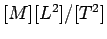, and the dimensions of velocity are. Hence, the dimensions of the left-hand side are, whereas the dimensions of the right-hand side are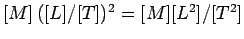. It follows that Eq. (4) is indeed dimensionally consistent. Thus,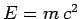holds good in mks units, in cgs units, in fps units, and in any other sensible set of units. Had Einstein proposed, or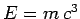, then his error would have been immediately apparent to other physicists, since these prospective laws are not dimensionally consistent. In fact,represents the only simple, dimensionally consistent way of combining an energy, a mass, and the velocity of light in a law of physics.

The last comment leads naturally to the subject of dimensional analysis: i.e., the use of the idea of dimensional consistency to guess the forms of simple laws of physics. It should be noted that dimensional analysis is of fairly limited applicability, and is a poor substitute for analysis employing the actual laws of physics; nevertheless, it is occasionally useful. Suppose that a special effects studio wants to film a scene in which the Leaning Tower of Pisa topples to the ground. In order to achieve this, the studio might make a scale model of the tower, which is (say) 1m tall, and then film the model falling over. The only problem is that the resulting footage would look completely unrealistic, because the model tower would fall over too quickly. The studio could easily fix this problem by slowing the film down. The question is by what factor should the film be slowed down in order to make it look realistic?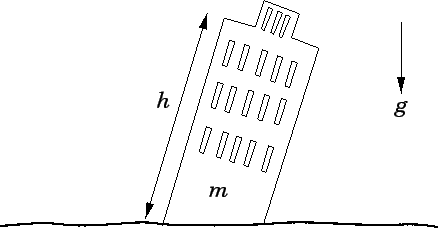Although, at this stage, we do not know how to apply the laws of physics to the problem of a tower falling over, we can, at least, make some educated guesses as to what factors the timerequired for this process to occur depends on. In fact, it seems reasonable to suppose thatdepends principally on the mass of the tower,, the height of the tower,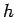, and the acceleration due to gravity,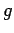. See Fig. 1. In other words,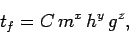(5)

whereis a dimensionless constant, and,, andare unknown exponents. The exponents,, andcan be determined by the requirement that the above equation be dimensionally consistent. Incidentally, the dimensions of an acceleration are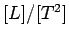. Hence, equating the dimensions of both sides of Eq. (5), we obtain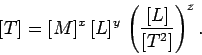(6)

We can now compare the exponents of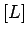,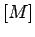, and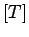on either side of the above expression: these exponents must all match in order for Eq. (5) to be dimensionally consistent. Thus,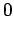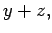(7)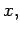(8)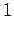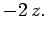(9)

It immediately follows that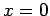,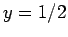, and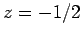. Hence,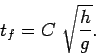(10)

Now, the actual tower of Pisa is approximately 100m tall. It follows that since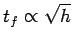(is the same for both the real and the model tower) then the 1m high model tower falls over a factor of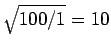times faster than the real tower. Thus, the film must be slowed down by a factor 10 in order to make it look realistic.Next: Worked example 1.1: Conversion Up: Introduction Previous: Precision and significant figures
Richard Fitzpatrick 2006-02-02